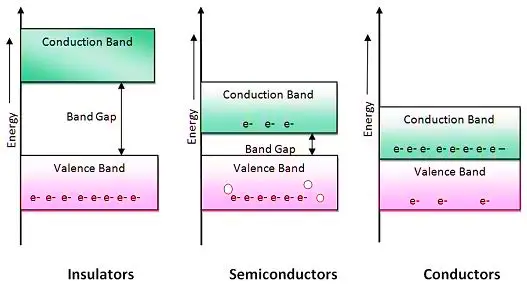## Search This Blog

In semiconductor physics we learnt about the types of semiconductors. In that study we known about the facts of conductivity in n-type and p-type semiconductors. For getting good understanding of mobility of electrons and holes we have to know about energy band diagram as explained below,

### Energy Band Diagram:The above diagram shows the energy band gap is how important in the physical properties of materials. when energy band gap is much large i.e grater than 7ev those are called as insulators. when it is around 0.7ev represents semiconductors. And when it is around null or negligible value then it is a conductor. So the amount of energy band gap differentiates the type of conductor also.

Before going to learn the concepts of diodes we may need to know how it is implemented by n-type and p-type semiconductors.

If energy gap is more the valence electrons are can't move from valence band to conduction band so we didn't have any conduction in insulators. As well in conductors we don't have the gap it is negligible so the conduction starts in less time. In semiconductors we have the time to start conduction.

Semiconductor materials are useful because their behavior can be easily manipulated by the addition of impurities, known as doping. Semiconductor conductivity can be controlled by the introduction of an electric or magnetic field, by exposure to light or heat, or by the mechanical deformation of a doped monocrystalline grid; thus, semiconductors can make excellent sensors. Current conduction in a semiconductor occurs via mobile or "free" electrons and holes, collectively known as charge carriers.

so, this is why we need semiconductors more than conductors in this growing world.

1.1.2.